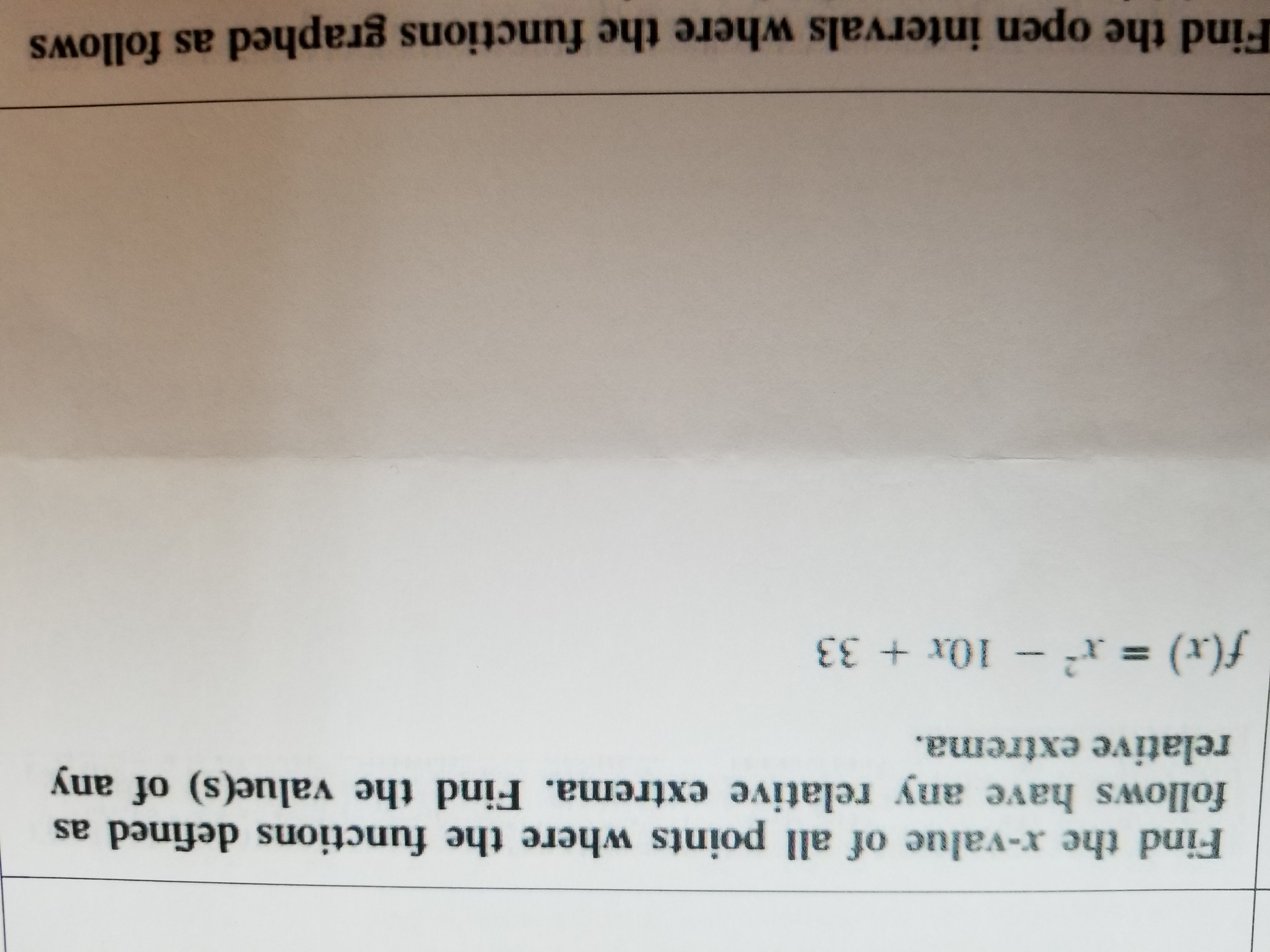# Find the x-value of all points where the functions defined asfollows have any relative extrema. Find the value(s) of anyrelative extrema.f(x)- 10x + 3:3Find the open intervals where the functions graphed as follows

Question
65 views

How do you find the x values and relative extrema.  Do you find the f'(x), then factor and use the critical points to find the relative extrema?help_outlineImage TranscriptioncloseFind the x-value of all points where the functions defined as follows have any relative extrema. Find the value(s) of any relative extrema. f(x)- 10x + 3:3 Find the open intervals where the functions graphed as follows fullscreen
check_circle

Step 1

First find the derivative of f(x) and compute the critical points.

Step 2

The second derivative of the function f(x)...

### Want to see the full answer?

See Solution

#### Want to see this answer and more?

Solutions are written by subject experts who are available 24/7. Questions are typically answered within 1 hour.*

See Solution
*Response times may vary by subject and question.
Tagged in

### Derivative